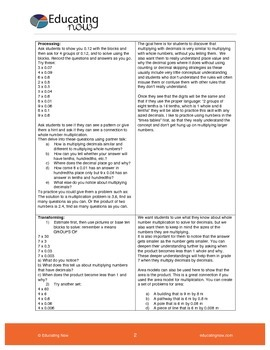# MULTIPLYING WHOLE NUMBERS BY DECIMALS USING BASE 10 BLOCKS PART 1Subject
Resource Type
Format
PDF (123 KB|5 pages)
Standards
\$0.99
\$0.99

### Description

My math lessons focus on student engagement and participation. I focus on developing conceptual understanding using manipulatives, specific language and activities.  My lesson plans also include differientiated tasks that cater to the varying levels in the classroom.

Goals:

1) To understand what it means to multiply whole numbers and decimals
2) To find the connection between multiplying whole numbers and decimal and whole numbers
3) To determine patterns of multiplying numbers with and without decimals in order to create efficient strategies for multiplying decimals

Rather than teaching students the procedure using rules such as “multiply as though they are whole numbers and then move the decimal back in”, we are going to explore what multiplication of a whole number by a decimal actually means. Our goal is to develop conceptual understanding and to build number sense.

Total Pages
5 pages
N/A
Teaching Duration
2 hours
Report this Resource to TpT
Reported resources will be reviewed by our team. Report this resource to let us know if this resource violates TpT’s content guidelines.

### Standards

to see state-specific standards (only available in the US).
Add, subtract, multiply, and divide decimals to hundredths, using concrete models or drawings and strategies based on place value, properties of operations, and/or the relationship between addition and subtraction; relate the strategy to a written method and explain the reasoning used.